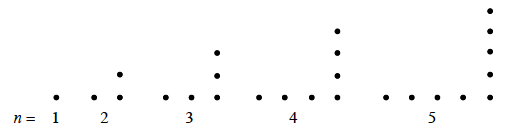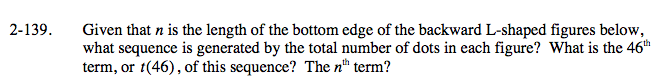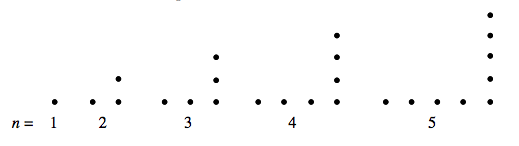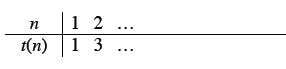Home > A2C > Chapter 2 > Lesson 2.2.2 > Problem2-139

2-139.

Given that n is the length of the bottom edge of the backward L-shaped figures below, what sequence is generated by the total number of dots in each figure? What is the 46th term, or t(46), of this sequence? The nth term? 2-139 HW eTool (Desmos). Homework Help ✎Start by finding the rule for the sequence.It may be helpful to copy and complete the table below.The sequence is odd numbers.

The 46th term = 91.

The nth term = 2n − 1

Explore what these answers mean.

Complete the table in the eTool below to solve the problem.
Click the link at right for the full version of the eTool: A2C 2-139 HW eTool